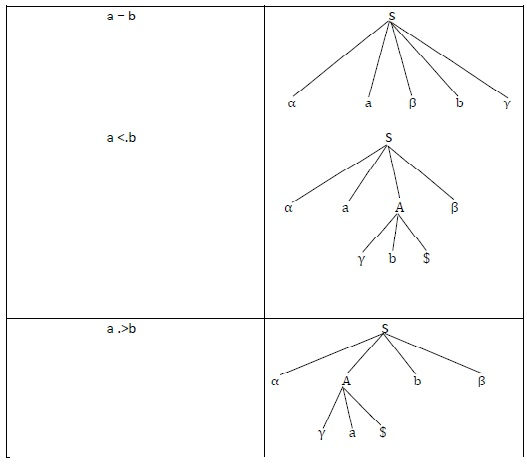# What are Precedence Relations in Operator Grammar?

For terminals a and b in an Operator Grammar we can have the following precedence Relations −

• a =. b(Equal Precedence) − If R.H.S of production is of form α a β b γ, where β can be ε or single non-terminal then a =. b.

Here, α and γ can be any strings.

Example − In grammar, S → m A c B e d

On Comparing mAcBed with αaβbγ

α = mA, a = c, β = B, b = e, γ = d

 Α A β b γ mA C B e d

So, comparing a with c and b with e we get c =.e.

We can also make a different combination for a and b.

In Grammar S → m A c Bed

α = ε, a = m, β = A, b = c, γ = Bed

 Α A β b γ Ε M A c Bed

So, comparing a with m and b with c

∴ m =. c

• a<.b (Less then)

If R.H.S of production is of form α a A β and A ⟹+ γb$where γ is ε or single nonterminal then a <.b. Example − In Grammar S → m A c D A → i On comparing m A c D with α a A β and A → i with A → γb$

 Α A A β Ε M A cD
 A → Γ b $A → E i ε ∴ α = ε, a = m, A = A, β = cD ∴ γ = ε, and b = i ∴ Applying the rule, a <. b means m <. i • a .> b (Greater Than) If R.H.S of production is of form αAbβ and A ⟹+ γa$ where $is ε or single nonterminal then a .> b. Example − In Grammar S → m A c D A → i On comparing m A cD with α a bβ and A → i with A → γa$

 Α A b β M A c D

 Α → Γ a $Α → E i ε ∴ α = m, A = A, b = c, β = D On comparing i with γa$

∴ γ = ε, a = i, \$ = ε

∴ On applying the rule,

a .> b means i .> c.

The Precedence relations between terminals symbols can also be shown by a Parse Tree −Algorithm for Computing Operator Precedence Relations

Input − An Operator Grammar

Output − A Precedence Relations between terminals and symbols.

Method

• begin
• For each production A → B1, B2, … … … . Bn

for i = 1 to n – 1

If Bi and Bi+1 are both terminals then

set Bi = Bi+1

If i ≤ n − 2 and Bi and Bi+2are both terminals and Bi+1 is non-terminal then

set Bi = Bi+2

If Biis terminal & Bi+1is non-terminal then for all a in LEADING (Bi+1)

set Bi <. a

If Biis non-terminal & Bi+1 is terminal then for all a in TRAILING (Bi)

set a . > Bi+1

• end